# PSAT Math : Translating Words to Linear Equations

## Example Questions

### Example Question #331 : Equations / Inequalities

We have three dogs: Joule, Newton, and Toby. Joule is three years older than twice Newton's age. Newton is Toby's age younger than eleven years. Toby is one year younger than Joules age. Find the age of each dog.

Joule: 8 years

Newton: 4 years

Toby: 8 year

Joule: 5 years

Newton: Not born yet

Toby: 1 year

none of these

Joule: 12 years

Newton: 1 year

Toby: 5 year

Joule: 9 years

Newton: 3 years

Toby: 8 year

Joule: 9 years

Newton: 3 years

Toby: 8 year

Explanation:

First, translate the problem into three equations. The statement, "Joule is three years older than twice Newton's age" is mathematically translated as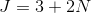where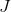represents Joule's age andis Newton's age.

The statement, "Newton is Toby's age younger than eleven years" is translated as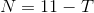where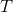is Toby's age.

The third statement, "Toby is one year younger than Joule" is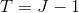.

So these are our three equations. To figure out the age of these dogs, first I will plug the third equation into the second equation. We get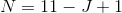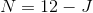Plug this equation into the first equation to get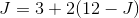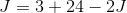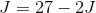Solve for. Add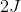to both sides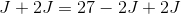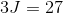Divide both sides by 3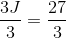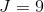So Joules is 9 years old. Plug this value into the third equation to find Toby's age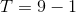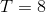Toby is 8 years old. Use this value to find Newton's age using the second equation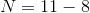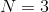Now, we have the age of the following dogs:

Joule: 9 years

Newton: 3 years

Toby: 8 years

### Example Question #1 : Solve Problems Leading To Two Linear Equations: Ccss.Math.Content.8.Ee.C.8c

Teachers at an elementary school have devised a system where a student's good behavior earns him or her tokens. Examples of such behavior include sitting quietly in a seat and completing an assignment on time. Jim sits quietly in his seat 2 times and completes assignments 3 times, earning himself 27 tokens. Jessica sits quietly in her seat 9 times and completes 6 assignments, earning herself 69 tokens. How many tokens is each of these two behaviors worth?

Sitting quietly is worth 9 tokens and completing an assignment is worth 3.

Sitting quietly is worth 3 tokens and completing an assignment is worth 7.

Sitting quietly and completing an assignment are each worth 4 tokens.

Sitting quietly is worth 3 tokens and completing an assignment is worth 9.

Sitting quietly is worth 7 tokens and completing an assignment is worth 3.

Sitting quietly is worth 3 tokens and completing an assignment is worth 7.

Explanation:

Since this is a long word problem, it might be easy to confuse the two behaviors and come up with the wrong answer. Let's avoid this problem by turning each behavior into a variable. If we call "sitting quietly"and "completing assignments", then we can easily construct a simple system of equations,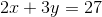and.

We can multiply the first equation byto yield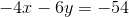.

This allows us to cancel theterms when we add the two equations together. We get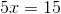, or.

A quick substitution tells us that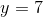. So, sitting quietly is worth 3 tokens and completing an assignment on time is worth 7.

### Example Question #2 : Solve Problems Leading To Two Linear Equations: Ccss.Math.Content.8.Ee.C.8c

Solve the following story problem:

Jack and Aaron go to the sporting goods store. Jack buys a glove for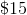andwiffle bats for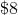each. Jack has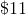left over. Aaron spends all his money onhats for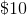each andjerseys. Aaron started with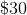more than Jack. How much does one jersey cost?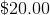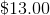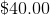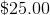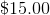Explanation:

Let's call "" the cost of one jersey (this is the value we want to find)

Let's call the amount of money Jack starts with ""

Let's call the amount of money Aaron starts with ""

We know Jack buys a glove forandbats foreach, and then hasleft over after. Thus: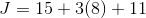simplifying,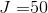so Jack started withWe know Aaron buyshats foreach andjerseys (unknown cost "") and spends all his money.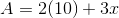The last important piece of information from the problem is Aaron starts withdollars more than Jack. So: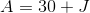From before we know: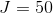Plugging in: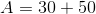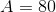so Aaron started with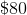Finally we pluginto our original equation for A and solve for x: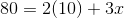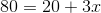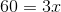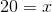Thus one jersey costs### Example Question #17 : Solve Problems Leading To Two Linear Equations: Ccss.Math.Content.8.Ee.C.8c

Read, but do not solve, the following problem:

Adult tickets to the zoo sell for $11; child tickets sell for$7. One day, 6,035 tickets were sold, resulting in $50,713 being raised. How many adult and child tickets were sold? Ifandstand for the number of adult and child tickets, respectively, which of the following systems of equations can be used to answer this question? Possible Answers: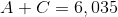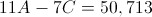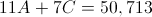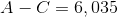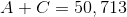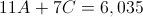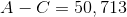Correct answer:Explanation: 6,035 total tickets were sold, and the total number of tickets is the sum of the adult and child tickets,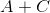. Therefore, we can say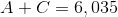. The amount of money raised from adult tickets is$11 per ticket mutiplied bytickets, or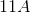dollars; similarly,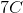dollars are raised from child tickets. Add these together to get the total amount of money raised: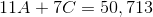These two equations form our system of equations.